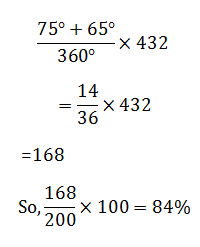# SSC Quantitative Aptitude Questions (Day-58)

Dear Aspirants, you can find the Quantitative Aptitude questions with detailed explanations for the SSC exams. Nowadays the competitive level of the exam has been increasing consistently. Due to the great demand for the government job, the level of the toughness reached greater. Candidates have to enhance the preparation process in order to drive in the right path. It doesn’t need to clear the prescribed cutoff. You must have to score good marks more than the cut off marks to get into the final provisional list. Here we have updating the Quantitative Aptitude questions with detailed explanations on a daily basis. You can practice with us and measure your level of preparation. According to that you can sculpt yourself in a proper way. SSC aspirants kindly make use of it and grab your success in your career.

Start Quiz

1)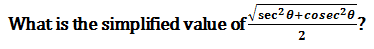(a) cosec 2θ

(b) sec2θ

(c) cosec θ sec θ

(d) tan θ

2)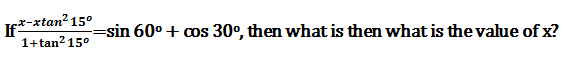(a) 2

(b) -1

(c) -2

(d) 1

3)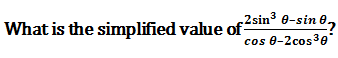(a) tan θ

(b) sin θ

(c) cos θ

(d) cot θ

4) If tan θ tan 5θ = 1, then what is the value of sin 2θ?

(a) 0

(b) 1/2

(c) 1√2

(d) √3/2

5) The angles of elevation of the top of a building from the top and bottom of a tree are 30° and 60° respectively. If the height of the tree is 50 m, thenwhat is the height of the building?

(a) 50√3

(b) 75

(c) 50(√3 + 1)

(d) 75√3

Direction (6-10): The given pie chart shows the marks obtained (in degrees) by a student in different subjects. The total marks obtained by the student in the examination is 432.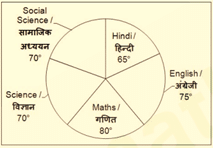6) What is the total of marks obtained in Hindi and Maths?

(a) 178

(b) 172

(c) 174

(d) 182

7) The marks obtained in science is what percentage of the total marks?

(a) 20.14

(b) 18.12

(c) 17.16

(d) 19.44

8) The marks obtained in Maths is how much percent more than the marks obtained in Social Science?

(a) 7.14

(b) 14.28

(c) 9.13

(d) 10.41

9) In how many subjects marks obtained are more than the average marks per subject?

(a) 3

(b) 1

(c) 2

(d) 4

10) If the maximum marks per subject is 100, then what is the total marks (in percentage) obtained in English and Hindi together?

(a) 168

(b) 68

(c) 76

(d) 84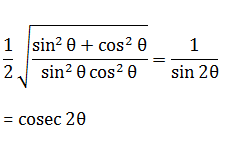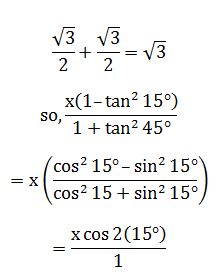= x × cos 30°

= √3/2 x = √3

⇒ x = 2when tan A . tanB= 1

Then A+B = 900

So, 6θ = 90°

or θ = 15°

∴ sin 30° = ½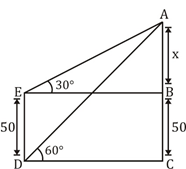From fig. EB = x√3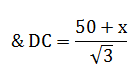But, EB = DC

⇒ 3x = 50 + x

⇒ x = 25

So, height of building = 75 m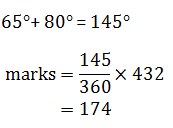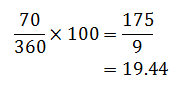10/70 × 100 = 14.28%

(360°)/5 = 72°

∴ Maths & English = 2.